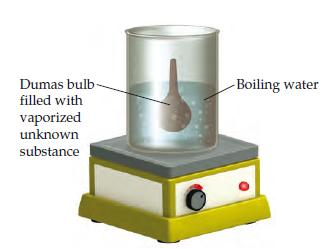# Problem: In the Dumas-bulb technique for determining the molar mass of an unknown liquid, you vaporize the sample of a liquid that boils below 100 oC in a boiling-water bath and determine the mass of vapor required to fill the bulb (see drawing ).From the following data, calculate the molar mass of the unknown liquid: mass of unknown vapor, 1.013 g ; volume of bulb, 354 cm3 ; pressure, 743 torr ; temperature, 99 oC .

###### FREE Expert Solution

Recall that molecular weight is in grams per 1 mole of a substance.

First, we have to calculate the amount of gas in moles using the ideal gas equation.

$\overline{){\mathbf{P}}{\mathbf{V}}{\mathbf{=}}{\mathbf{n}}{\mathbf{R}}{\mathbf{T}}}$

P = pressure, atm
V = volume, L
n = moles, mol
R = gas constant = 0.08206 (L·atm)/(mol·K)
T = temperature, K

Isolate n (number of moles of gas):

93% (93 ratings)###### Problem Details

In the Dumas-bulb technique for determining the molar mass of an unknown liquid, you vaporize the sample of a liquid that boils below 100 oC in a boiling-water bath and determine the mass of vapor required to fill the bulb (see drawing).

From the following data, calculate the molar mass of the unknown liquid: mass of unknown vapor, 1.013 g ; volume of bulb, 354 cm3 ; pressure, 743 torr ; temperature, 99 oC .